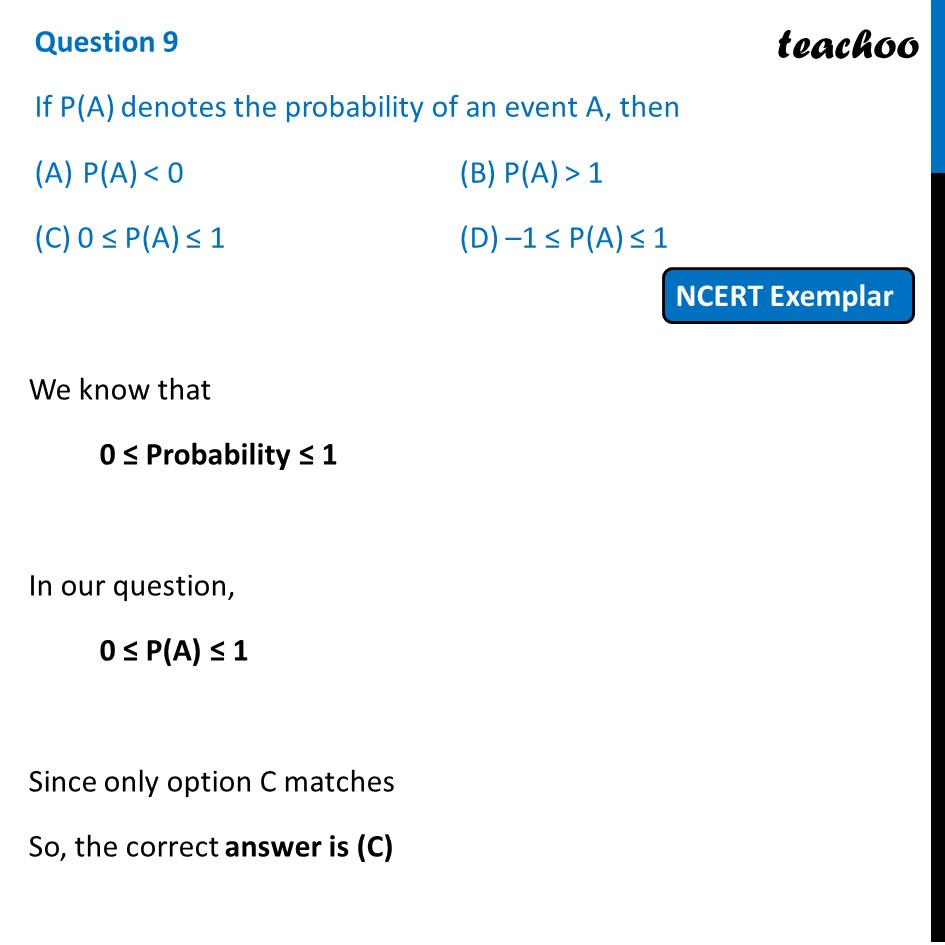NCERT Exemplar - MCQ

Chapter 14 Class 10 Probability
Serial order wise

## (D) –1 ≤ P(A) ≤ 1Learn in your speed, with individual attention - Teachoo Maths 1-on-1 Class

### Transcript

Question 9 If P(A) denotes the probability of an event A, then P(A) < 0 (B) P(A) > 1 (C) 0 ≤ P(A) ≤ 1 (D) –1 ≤ P(A) ≤ 1 We know that 0 ≤ Probability ≤ 1 In our question, 0 ≤ P(A) ≤ 1 Since only option C matches So, the correct answer is (C)